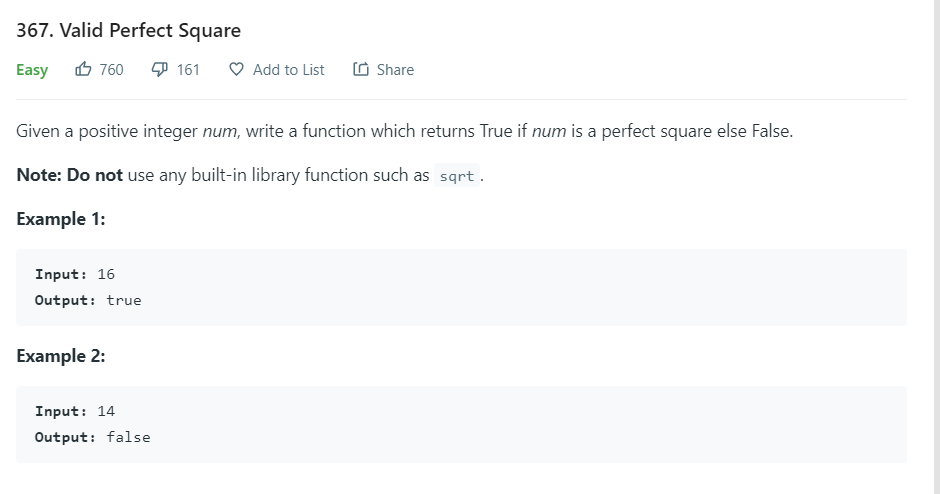# Solution to Leetcode’s Valid Perfect SquareValid perfect square description. Leetcode 367

## THE NAIVE APPROACH

Of course the naive or brute force approach would be to loop through from 1 to the number, multiplying a number by itself and checking if the product equals the number you are looking for. if it does, then return true, else return false.

`public static boolean isPerfectSquareWithBruteForce(int num) {    if (num == 1) return true;    for (int i = 1; i < num; i++) {        if (i*i == num) return true;    }    return false;}`
`public static boolean isPerfectSquareWithHalfNumber(int num) {    if (num ==  1 || num ==  0) return true;    for (int i = 1; i < num/2; i++ ) {        if (i*i == num) return true;    }    return false;}`

## A FASTER SOLUTION

A better way to solve this problem would be to use Binary Search. This solves the problem by using the divide and conquer methodology. It keeps splitting the number into half and checking if number is found.

`public static boolean isPerfectSquareWithBinarySearch(int num) {    double start = 1;    double end = num/2;    if (num == 1) return true;    while (start <= end) {        double mid = Math.floor((end - start)/2) + start;        if (mid * mid ==  num) {            return true;        }else if (mid * mid < num) {            start = mid + 1;        }else {            end = mid - 1;        }    }    return false;}`

--

--